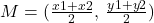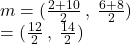## Find the midpoint of the line segment with the endpoints A and B. A(2,6); B(10,8) whats the midpoint of the segment?

Question

Find the midpoint of the line segment with the endpoints A and B.
A(2,6); B(10,8)
whats the midpoint of the segment?

in progress 0
5 months 2021-08-15T00:30:04+00:00 1 Answers 6 views 0

## ( 6 , 7 )

Step-by-step explanation:

The midpoint M of two endpoints of a line segment can be found by using the formulaFrom the question we haveWe have the final answer as

### ( 6 , 7 )

Hope this helps you